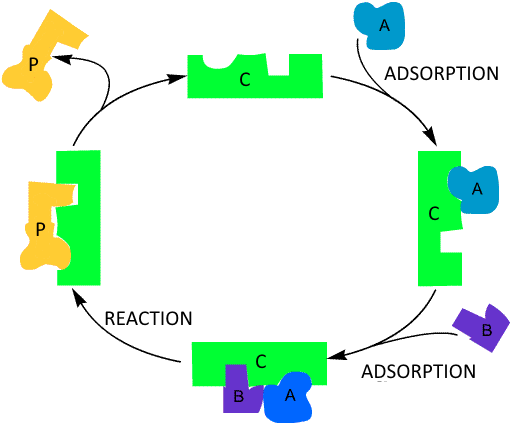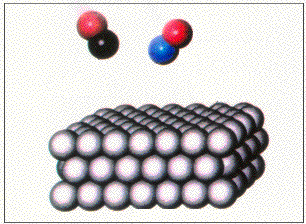Search:

# The heterogeneous catalysis

## Definition

The heterogeneous catalysis involves the use of a catalyst in a different phase from the reactants.

## Frequent caseOften reagents (here $A$ and $B$) are adsorbed on a solid catalyst ($C$) and there they combine to form the product(s) ($P$) that is (are) liberated later:

## Example

In catalytic converters for cars a reaction (among others) is the conversion of the toxic gases nitrous oxide and carbon monoxide into carbon dioxide and nitrogen: $2\;NO$ $+$ $2\;CO$ $\longrightarrow$ $2\;CO_2$ $+$ $N_2$ This reaction is accelerated on a solid catalyst, the rhodium metal. The mechanism seems to be the following:

Click on the image !